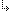- 4.45.2.45. -4. Standard Units4.45. Math - Extended Mathematics unit4.45.2. Math Unit Procedures and Functions4.45.2.45. Modf function

## 4.45.2.45. Modf function

Targets: MS-DOS, OS/2, Win32

Math Unit

Splits a floating-point value into fractional and integer parts.

Declaration:
```function Modf(X: Extended; var Y: Longint): Extended;
function Modf(X: Extended; var Y: Integer): Extended;```
Remarks:

The Modf function breaks down the floating-point value X into fractional and integer parts, each of which has the same sign as X.

The signed fractional portion of X is returned. The integer portion is stored as a floating-point value at Y.

- 4.45.2.45. -